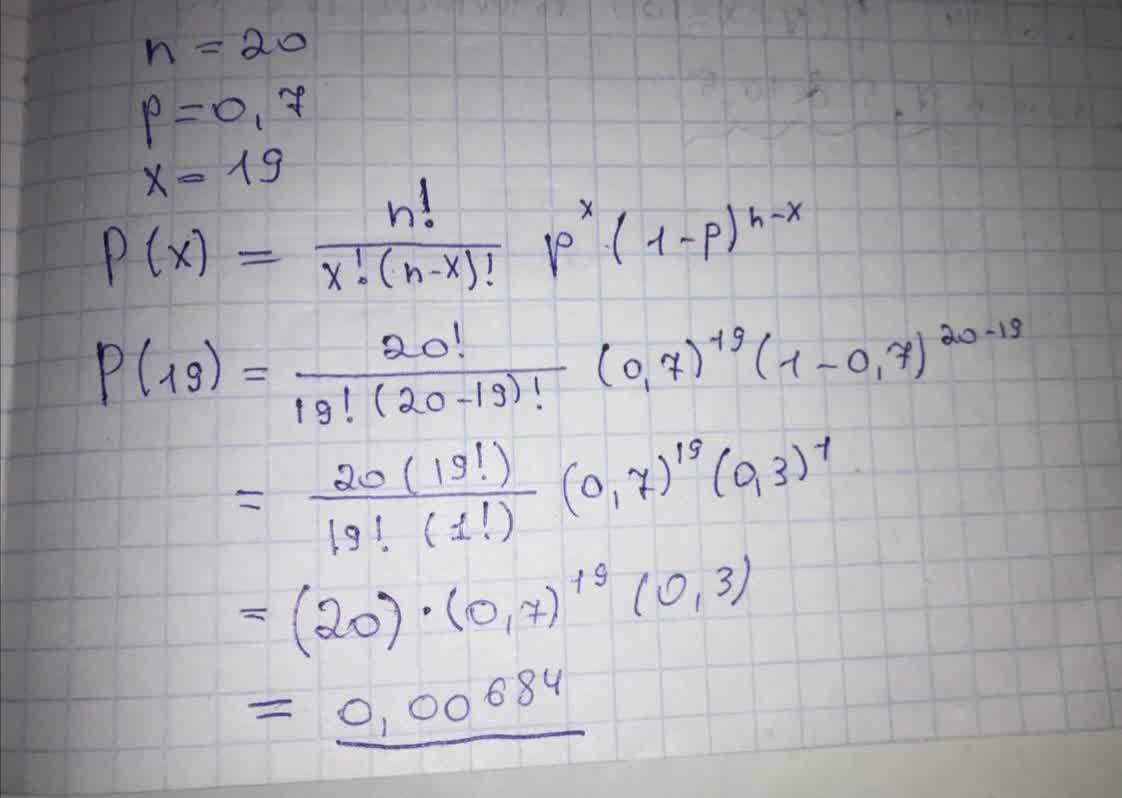# A binomial probability experiment is conducted with the given parameters. Compute the probability of x successes in the n independent trialspreprekomW 2021-08-30 Answered
A binomial probability experiment is conducted with the given parameters. Compute the probability of x successes in the n independent trials of the experiment. n=20​, p=0.7​, x=19 P(19)=
You can still ask an expert for help

• Questions are typically answered in as fast as 30 minutes

Solve your problem for the price of one coffee

• Math expert for every subject
• Pay only if we can solve itopsadnojD
On photo: